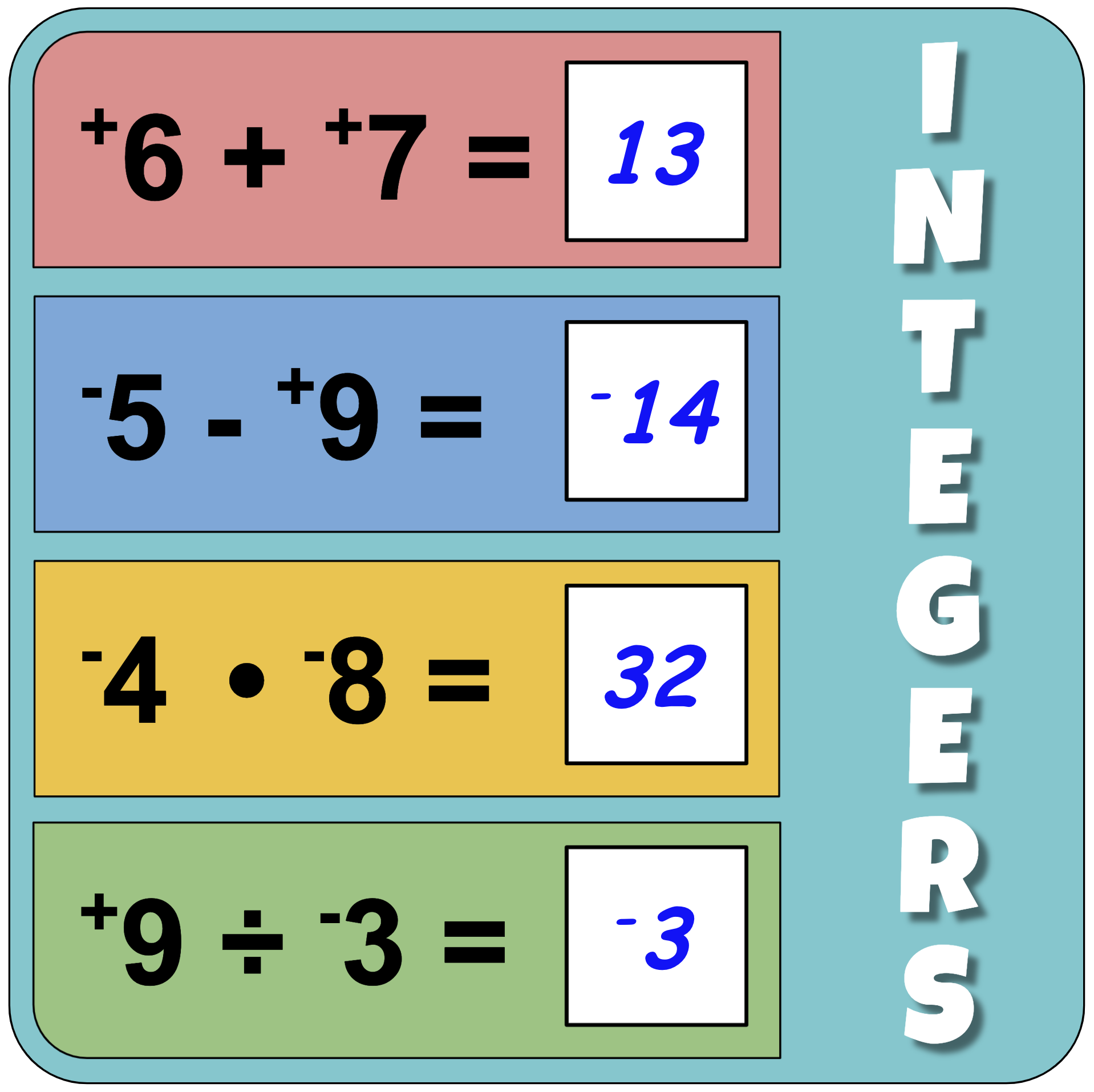Back to Mr. Anker TestsUse these practice activities to help you better understand integers.

Teachers & Parents: It is recommended that students complete and master
each activity before moving on to the next.

 Integers 1AdditionAdding one-digit integers. Integers 2AdditionAdding one and two-digit integers. Integers 3AdditionAdding integers with greater +/- values. Integers 4AdditionAdding integers with even greater +/- values.
 Integers 5SubtractionSubtracting one-digit integers. Integers 6SubtractionSubtracting one and two-digit integers. Integers 7SubtractionSubtracting integers with greater +/- values. Integers 8SubtractionSubtracting integers with even greater +/- values.
 Integers 9MultiplicationMultiplying one-digit integers. Integers 10MultiplicationMultiplying one and two-digit integers. Integers 11MultiplicationMultiplying integers with greater +/- values. Integers 12MultiplicationMultiplying integers with even greater +/- values.
 Integers 13DivisionDividing one-digit integers. Integers 14DivisionDividing one and two-digit integers. Integers 15DivisionDividing integers with greater +/- values. Integers 16DivisionDividing integers with even greater +/- values.
 Integers 17MixedAll four operations with one-and two digit integers. Integers 18MixedAll four operations with one and two-digit integers. Integers 19MixedAll four operations & grouping with integers. Integers 20MixedAll four operations & grouping with integers.